ChemTalk

# Quantized Energy## Core Concepts

In this article, you will learn about how energy is quantized, the basis of modern quantum mechanics! You will also learn about a few of the scientists who helped further our understanding of quantization, the photoelectric effect, and how quantization can be used to explain atomic absorption and emission spectra. The quantization of energy helps us explain why atoms are stable, how they give off and absorb light, and how chemical bonds form.

## What does “quantized energy” mean?

Max Planck was the first to postulate that energy was quantized and could be radiated or absorbed only in multiples of a small unit of energy, known as a quantum. He used this smallest unit to create Planck’s constant, or h, a variable representing a minimum amount of energy that can be transferred in a quantum of electromagnetic radiation. The value of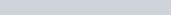.

The analogy commonly used is climbing a staircase, where each step represents a specific energy level. In the world of chemistry, energy is not continuous; instead, it exists in fixed amounts. Electrons in atoms can only occupy certain steps on the energy staircase, as they can only have specific energy levels. When an electron gains or loses energy, it jumps to a higher or lower step, respectively.The ladder on the left represents how energy is quantized, or restricted to certain levels. Think of how when you climb a ladder you have to use the rungs; you cannot step between the rungs to climb. On the other hand, when climbing the ramp on the right, you can stop at any point, meaning it is continuous.

## The Photoelectric Effect and Quantized Energy

The photoelectric effect was a phenomenon observed by Heinrich Hertz and further studied by Einstein. It proved Planck’s observation that light’s energy was quantized. Before the discovery of the photoelectric effect by Einstein, light was believed to be solely a wave, which, according to classical physics, would have meant that it could have any energy value.

When light strikes certain metals, electrons are emitted from their surface. Einstein explained this phenomenon by introducing “photons,” or energy packets of light. Each photon transfers its energy to an electron, freeing it from the metal. If a photon’s energy isn’t enough, no emission occurs, no matter how much the intensity of the light is raised. Thus, even with a brighter light, if the energy/frequency is insufficient there will be no electrons emitted from the metal.

This demonstrated that energy is quantized by showing that the emission of electrons from a metal’s surface by light depends on the frequency / color of the light rather than its intensity.Light of a sufficient frequency hits a metal plate and knocks electrons off.

#### Mathematics of the Photoelectric Effect

There are some equations related to the photoelectric effect which can allow you to calculate if light is of sufficient energy to knock electrons off the metal. One such equation is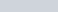. This equation can be used to calculate the energy of a single photon given its frequency. The unit of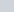, energy, is joules,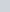is Planck’s constant, and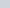is the frequency in Hz. Importantly, light energy does not depend on intensity, which can be thought of as the height or amplitude of the light wave.

In this equationcan be substituted with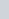, where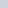is the speed of light,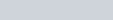, andis the wavelength in meters. This substitution gives the equation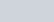.

The minimum amount of energy required to eject an electron from a metal surface is called its work function. There are three possible cases for whether an electron is knocked off or not depending on the energy of the photons of the light:

• If the energy of the photons is too low, the work function isn’t met, meaning no electrons will be ejected.
• If the work function is met, but the energy is equal to the work function, the electron will be ejected. However, the electron will have no extra kinetic energy.
• If the energy of the photons exceeds the work function, the extra energy will contribute to the kinetic energy of the emitted electron.

## Quantized Energy explains Absorption and Emission Spectra

In atomic emission spectra, atoms absorb certain wavelengths of energy and release it as light, producing distinct colored lines. The opposite is an atomic absorption spectra, where atoms absorb specific wavelengths of light, leaving dark lines in the spectrum.

Electrons can move to higher energy levels when absorbing photons, or move to lower energy levels. In either case the electron transition emits or absorbs a photon with a specific energy. This process leads to the distinct lines that can be seen in emission and absorption spectra. These lines can be explained using the Bohr model, a model of an atom where electrons travel in distinct orbits/shells around the nucleus. Each line in an element’s emission spectrum represents the color of photons emitted when an electron jumps between electron shells.

For example, take a look at the emission spectra for the element Neon below. In particular, notice how most of the emitted frequencies are in the yellow-red range.

This lines up with what we see in real life! If you’ve ever seen neon lights they glow a shade of orange. And if you’ve seen similar tube lights in other colors, those are different elements. Each element has a different emission spectra that allow them to glow a different color.